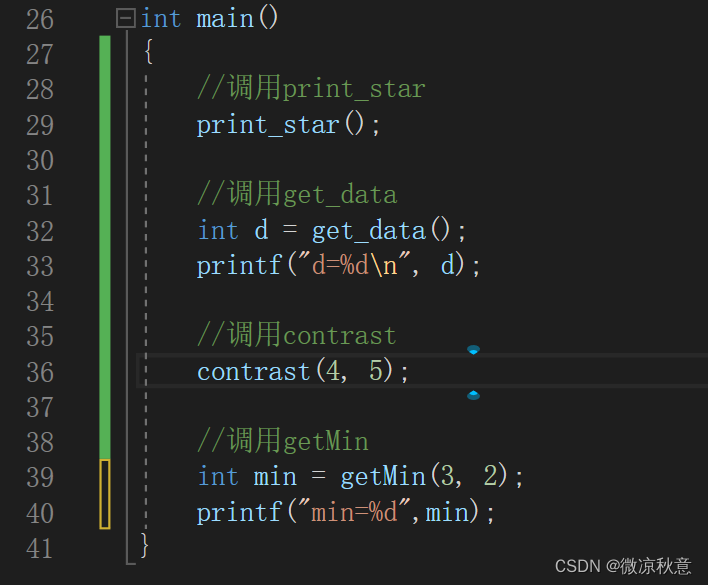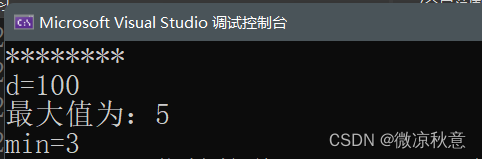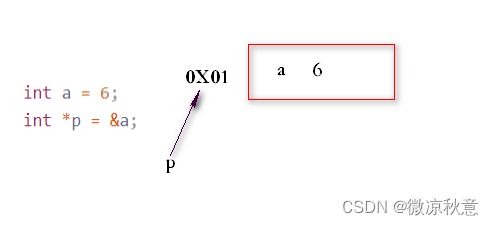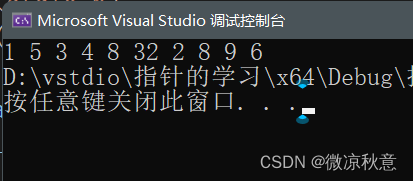0

# 【C语言】规范掌握C语言函数|数组名的妙用|指针快速入门|综合使用小案例

✅作者简介：热爱后端语言的大学生，CSDN内容合伙人
✨精品专栏：C++面向对象

### 文章目录

🔥前言

C语言计算机二级考试快开始了，为了给我对象加油助力，我准备总结一下C语言中函数、数组、指针的知识点并设计三种综合案例作为练习巩固。

## 1、函数的结构

C语言要求，在程序中用到的所有函数必须先定义，后使用。那么怎么去定义一个函数呢？

1. 指定函数的类型，即返回值类型，这个类型可以是整型、浮点型、字符型甚至是自定义类型
2. 指定函数的名字，取名尽量按照好理解的意义来做，方便后续的调用
3. 指定函数的参数列表，可以无参数，有参数的时候注意定义好参数的类型以及名字
4. 指定函数完成特定功能，这个在函数体内定义
5. 函数可以事先声明，跟函数定义不同的是以分号结尾

``````intMax(int x,int y);intMax(int x,int y){return x>y?x:y;}
``````

``````int
``````

``````Max
``````

``````(int x,int y)
``````

``````return
``````

``````void
``````

## 1.1、无参无返

**返回值类型为

``````void
``````

，参数列表为空**

``````voidprint_star(){printf("********\n");}
``````

## 1.2、无参有返

**返回值类型设置为

``````int
``````

，return 的值也是整型**

``````intget_data(){int data =100;return data;}
``````

## 1.3、有参无返

``````a
``````

``````b
``````

，调用该函数就能自动打印出二者中的较大值

``````voidcontrast(int a,int b){int c =0;if(a > b)
c = a;else
c = b;printf("最大值为：%d", c);}
``````

## 1.4、有参有返

``````getMin
``````

``````intgetMin(int a,int b){return a < b ? a : b;}
``````

## 1.5、主函数中的调用及运行效果## 2、C语言数组创建和基本操作

• 数组的地址在一段内存空间中连续
• 数组中的每一个元素都属于同一个数据类型
• 数组从零开始计数，不能访问越界元素
• 数组名代表该数组的首地址- 因此数组除了写成：`int A`; 还可以写成：`int *A`- 这两种定义方式下，数组名A都代表着地址

## 2.1、数组创建的方式

``````//1、直接创建，不初始化，要给数组确定的大小int A;//2、创建时赋值，使用大括号，此时可省略中括号的值，例如：int B[]={3,1,2};int B={3,2,1};//3、全部初始化为0int C={0};
``````

``````//1、不初始化，规定好行数和列数int A;//2、全部初始化为0int B={0};//3、给特定部分赋值，这里的行的大小可以省略，因为编译器可以根据赋值情况推断出有四行数据int C[]={{0},{1},{1,2,3,4,5},{3}};
``````

## 2.2、数组元素的引用

``````int A={1,3,2,5,4};for(int i=0;i<5;i++){printf("%d ",A[i]);}//打印结果：1 3 2 5 4
``````

``````int A=66;//此时再访问结果就变为了66
``````

**这里唯一要注意的就是不要越界访问，访问

``````A
``````

``````A
``````

**

## 2.3、有关数组名的干货小知识

``````sizeof
``````

``````int A[]={1,5,3,5,8,4,3};int len =sizeof(A)/sizeof(A);//代码执行结果为7
``````
``````sizeof
``````

``````int A;int i =0;while(i <5){scanf("%d", A +(i++));}
``````

``````scanf
``````

``````&
``````

• 当i为0时，`A+(i++)`的结果还是A，而A又代表数组的首地址，那么这个数据就赋给了A
• 上一步经过`i++`，之后i为1，A+(i++)的结果是`A+1`，而地址的+1不是加一个字节，而是一块内存，在这个数组里就是+`4`，对应A的位置。因此第二个数据赋值给了`A`
• 以此类推，当i为5时，`A+4`对应的位置`A`也完成了赋值，i<5不成立，循环结束，数组完成赋值。

## 3.1、指针的定义和使用

``````int a =6;int*p =&a;
``````

``````int a =6;int*p;
p =&a;
``````

``````p
``````

``````a
``````

``````*p
````````````0X01
``````

``````*p
``````

## 3.2、指针所占内存空间

``````sizeof
``````

``````int a =66;double b=1.0;printf("%d",sizeof(a));//结果为4printf("%d",sizeof(b));//结果为8
``````

*不仅如此，

``````sizeof
``````

``````printf("%d",sizeof(int));//4printf("%d",sizeof(float));//4printf("%d",sizeof(double));//8printf("%d",sizeof(char));//1
``````

``````printf("%d",sizeof(int*));//8printf("%d",sizeof(double*));//8printf("%d",sizeof(char*));//8
``````

## 3.3、空指针和野指针

``````int*p =NULL;
``````
• 空指针用于给指针变量进行初始化
• 空指针是不可以进行访问

1. 指针未初始化 - 跟空指针类似
2. 指针越界访问 - 例如：用指针遍历数组时超过了数组的边界
3. 指针释放后未置空 - 只是将指针`free`掉了，未将NULL赋值给该指针

## 4.1、指针和函数小案例

``````voidswap(int* a,int* b){int temp =*a;*a =*b;*b = temp;}intmain(){int a =10, b =20;swap(&a,&b);printf("a=%d",a);printf("b=%d",b);}
``````

## 4.2、指针和数组小案例

``````#include<stdio.h>intmain(){int arr[]={1,5,3,4,8,32,2,8,9,6};int len =sizeof(arr)/sizeof(arr);//计算数组长度int* p = arr;//p指向数组首地址for(int i =0; i <len; i++){printf("%d ",*p);//p是地址，*p才是地址对应的值
p++;}//p++意为p指针向后偏移四个字节，因为数组中地址连续，所以就是下一个数组的元素}
``````## 4.3、数组和函数小案例

``````voidsetArr(int A[],int len){for(int i =0; i < len; i++){scanf("%d",&A[i]);}printf("赋值已经就绪！\n");}
``````

``````voidgetArr(int*A,int len){printf("打印数组中的所有元素：\n");for(int i =0; i < len; i++){printf("%d ", A[i]);}}
``````

``````int A[]
``````

``````int *A
``````

，二者本质都是一样的。

``````那么此篇博客就到此结束了，二级考试将至，希望大家都能蟾宫折桂，取得佳绩！
``````

### “【C语言】规范掌握C语言函数|数组名的妙用|指针快速入门|综合使用小案例”的评论:

##### 关于作者##### overfit同步小助手